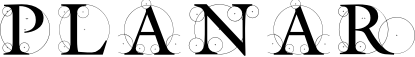#### Previous topic

planar – Global Definitions

#### Next topic

planar.Vec2Array – 2D Vector Arrays

# planar.Vec2 – 2D Vectors¶

class planar.Vec2

Two dimensional immutable vector.

Parameters: x (float) – x coordinate. y (float) – y coordinate.
almost_equals(other)

Compare vectors for approximate equality.

Parameters: other (Vec2) – Vector being compared. True if distance between the vectors < EPSILON.
angle_to(other)

Compute the smallest angle from this vector to another.

Parameters: other (Vec2) – Vector to compute the angle to. Angle in degrees in the range (-180, 180]. float
clamped(min_length=None, max_length=None)

Compute a vector in the same direction with a bounded length. If min_length <= self.length <= max_length then the original vector is returned.

Parameters: min_length (float) – Minimum length of computed vector. Note if the input vector is null, the null vector is always returned. max_length (float) – Maximum length of computed vector. Must be >= min_length. Vec2
cross(other)

Compute the cross product with another vector.

Parameters: other (Vec2) – The vector with which to compute the cross product. The length of the cross-product vector float
distance_to(other)

Compute the distance to another point vector.

Parameters: other (Vec2) – The point vector to which to compute the distance. float
dot(other)

Compute the dot product with another vector.

Parameters: other (Vec2) – The vector with which to compute the dot product. float
lerp(other, bias)

Compute a vector by linear interpolation between this vector and another.

Parameters: other (Vec2) – The vector to interpolate to. its value is returned when bias == 1.0. bias (float) – Interpolation value when in the range [0, 1]. Becomes an extrapolation value outside this range. Vec2
normalized()

Return the vector scaled to unit length. If the vector is null, the null vector is returned.

Return type: Vec2
perpendicular()

Compute the perpendicular vector.

Return type: Vec2
classmethod polar(angle, length=1.0)

Create a vector from polar coordinates.

Parameters: angle (float) – Vector angle in degrees from the positive x-axis. length (float) – The length of the vector. Vec2
project(other)

Compute the projection of another vector onto this one.

Parameters: other (Vec2) – The vector to project. Vec2
reflect(other)

Compute the reflection of this vector against another.

Parameters: other (Vec2) – The vector to reflect against. Vec2
rotated(angle)

Compute the vector rotated by an angle.

Parameters: angle (float) – The angle to rotate by, in degrees. Vec2
scaled_to(length)

Compute the vector scaled to a given length. If the vector is null, the null vector is returned.

Parameters: length (float) – The length of the vector returned, unless the vector is null. Vec2
angle

The angle the vector makes to the positive x axis in the range (-180, 180].

is_null

Flag indicating if the vector is effectively zero-length.

Returns: True if the vector length < EPSILON.
length

The length or scalar magnitude of the vector.

length2

The square of the length of the vector.

x

The horizontal coordinate.

y

The vertical coordinate.

class planar.Point

An alias for Vec2. Useful for code clarity.